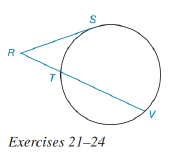Chapter 6.3, Problem 21EElementary Geometry For College St...

7th Edition
Alexander + 2 others
ISBN: 9781337614085

Solutions

Chapter
SectionElementary Geometry For College St...

7th Edition
Alexander + 2 others
ISBN: 9781337614085
Textbook Problem

In the figure for Exercises 21 to 24, R S ¯ is tangent to the circle at S. See Theorem 6.3.7.Given: R S = 8     a n d     R V = 12 Find: RT.To determine

To find:

To find RT.

Explanation

Given that, RS=8andRV=12.

The diagrammatic representation is given below,

Theorem:

If a tangent segment and a secant segment are drawn to a circle from an external point, then the square of the length of the tangent equals the product of the length of the secant with the length of its external segment.

By using the theorem to get the following,

RS2=RVRT

We know that

RV=RT+TV

Substitute the values RS=8

Still sussing out bartleby?

Check out a sample textbook solution.

See a sample solution

The Solution to Your Study Problems

Bartleby provides explanations to thousands of textbook problems written by our experts, many with advanced degrees!

Get Started

Prove that abx2dx=b3a33.

Single Variable Calculus: Early Transcendentals, Volume I

Fill in each blank: 6000floz=gal

Elementary Technical Mathematics

limx6(x210x)=. a) 24 b) 6 c) 26 d) does not exist

Study Guide for Stewart's Single Variable Calculus: Early Transcendentals, 8th

A normal vector to the plane 2x + 3y + 6z =–20 is:

Study Guide for Stewart's Multivariable Calculus, 8th